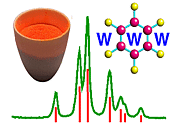Generation of hkl, d, and 2θ Values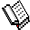Course Material Index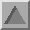Section Index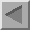Previous Page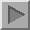Next Page

Generation of hkl, d, and 2θ Values

It is frequently very useful in the analysis of powder diffraction data to be able to calculate a set of hkl values, d spacings, and equivalent 2θ values from a Bravais lattice of given unit-cell dimensions.

A web-based program, identified by the clickable icon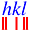, is provided here to demonstrate the calculation of hkl values and d spacings. You can access the program by clicking on the icon. We shall be making use of this program in this and other sections of the course. Occasionally, the values will be preset by us, and under these circumstances, you will only need to click the submit button and nothing else. The remainder of this page provides an explanation as to how this type of program functions in practice.

In order to generate our d spacings we need to consider the two end limits dmin and dmax corresponding to minimum and maximum d spacing values. The maximum d spacing is simply determined by the size of the unit cell and therefore has a predetermined maximum limit. By contrast, the minimum d spacing tends towards zero, with the limit of zero corresponding to an infinite number of lattice planes. However, for angle-dispersive diffraction a practical limit on observable d spacings is provided by the radius of the Ewald sphere, i.e. diffraction cannot be observed for scattering angles beyond 180°. Experimentally, a maximum scattering angle well-below 180° will place a higher limit on the value of dmin.

The minimum d spacing and the lengths of the unit cell, a, b, c, determine the minimum and maximum values for the indices hkl as follows:

 hmin = - a / dmin and hmax = a / dmin kmin = - b / dmin and kmax = b / dmin lmin = - c / dmin and lmax = c / dmin

A computer program can loop through all permutations of hkl within these limits and generate d spacings using the metric tensor equation given previously.

Application of Symmetry

The method described above is fine for triclinic Bravais lattices, but is unsuitable for the higher symmetry systems where many reflections will be symmetry equivalent. In practice, it is only necessary to generate the unique hkl values and their corresponding multiplicity value, j, (as discussed earlier). A brief explanation of the application of symmetry to hkl generation is provided below.

Consider, for example, the cubic crystal system, where the d spacings are calculated according to the equation:

1/d2 = A (h2 + k2 + l2).

It can be seen from this equation that the d spacing for the 321 reflection will be the same as for, say, the 213 or 312 reflections for cubic symmetry. There is little point in generating hkl values for reflections that are equivalent by symmetry, so extra conditions are place on the minimum and maximum values of the indices hkl. Some typical examples are given in the table below:

Crystal SystemLaue ClassLimits
Cubic m-3m hmin = 0,   kmin = 0 and kh,   lmin = 0 and lk
m-3 hmin = 0,   kmin = 0 and kh,   lmin = 0 and lh
Tetragonal 4/mmm hmin = 0,   kmin = 0 and kh,   lmin = 0
4/m hmin = 0,   kmin = 0,   lmin = 0
Orthorhombic mmm hmin = 0,   kmin = 0,   lmin = 0
Monoclinic 2/m kmin = 0,   lmin = 0

From the above table, it can be seen that for monoclinic symmetry (with y as the unique axis) reflection indices will be generated for +ve values only of k and l, but with both -ve and +ve values of h. In practice a few other boundary conditions are also applied, but a full description of these lies beyond the scope of this course.

The second application of symmetry concerns centred lattices. A centred lattice has fewer reflections than a primitive one, and it is useful to be able to eliminate reflections due to centred unit cells. A full discussion on this subject will be given in the fourth section on symmetry, but you should note at this point that the lattice centring of the Bravais lattice can be specified when generating reflection indices.Course Material IndexSection IndexPrevious PageNext Page
 © Copyright 1997-2006.  Birkbeck College, University of London. Author(s): Jeremy Karl Cockcroft